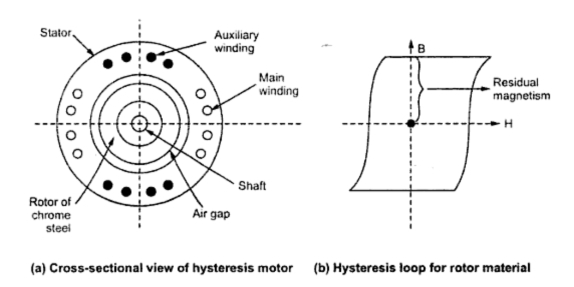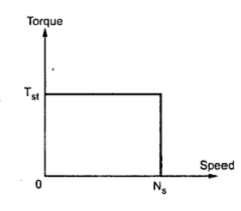### Hysteresis Motor

This is the synchronous motor which does not require and d.c. excitation to the rotor and it uses non projected poles.
It consists of a stator which carries main and auxiliary windings so as to produce rotating magnetic field. The stator can also be shaded pole type. The rotor is smooth cylindrical type made up of hard magnetic material like chrome steel or alnico for high retentivity. This requires to select a material with high hysteresis loop area. The rotor does not carry any winding. The construction is shown in the Fig. 1(a) while nature of hysteresis loop require for rotor material is shown in the Fig., 1(b).Fig.1

When stator is energized, it produces rotating magnetic field. The main and auxiliary, both the windings must be supplied continuously at start as well as in running condition so as to maintain the rotating magnetic field. This field induces pole in the rotor. The hysteresis phenomenon is dominate for the rotor material chosen and due to which rotor pole axis lag behind the axis of rotating magnetic field. Due to this, rotor poles get attracted towards the moving stator field poles. Thus rotor gets subjected to torque called hysteresis torque. This is torque is constant at all speeds.
When the stator field axis moves forward, due to high retentivity the rotor pole strength remains maintained. So higher the retentivity, higher is the hysteresis torque.
Initially rotor starts rotating due to combined effect of hysteresis torque as well as torque due to eddy currents induced in the rotor. Once the speed is near about the synchronous, the stator pulls rotor into synchronism. In such case, as relative motion between stator field and rotor vanishes, so the torque due to eddy current vanishes. Only hysteresis torque is present which keeps rotor running at synchronous speed. The high retentivity, ensures the continuous magnetic either rotates at synchronous speed or not at all.
1.1 Mathematical Analysis
The eddy current loss in the machines is given by,
P= Ke f22 B2
where  Ke  = Eddy current constant
f= Frequency of eddy currents
B = Flux density
We know the relation between rotor frequency fand supply frequency f1 .
f2  = sf1
where   s = Slip
P= Ke s2  f12 B2
The torque due to eddy currents is given by

So   T  α  s             ..All other parameters are constant
So when rotor rotates at synchronous speed, the slip becomes zero and torque due to eddy current component vanishes. It only helps at start.
The hysteresis loss is given by,
P = K f2  B1.6
= K s f1 B1.6
The corresponding torque is given by,

Note : Thus the hysteresis torque component is constant at all the rotor speeds.
1.2 Torque-Speed Characteristics
The staring torque and running torque is almost equal in this type of motor. As stator carries mainly the two windings its direction can be reversed by interchanging the terminals of either main winding or auxiliary winding. The torque-speed characteristics is as shown in the Fig. 2.Fig. 2  Torque-speed characteristics of hysteresis motor

As seen from the characteristics torque at start is almost throughout the operation of the motor.